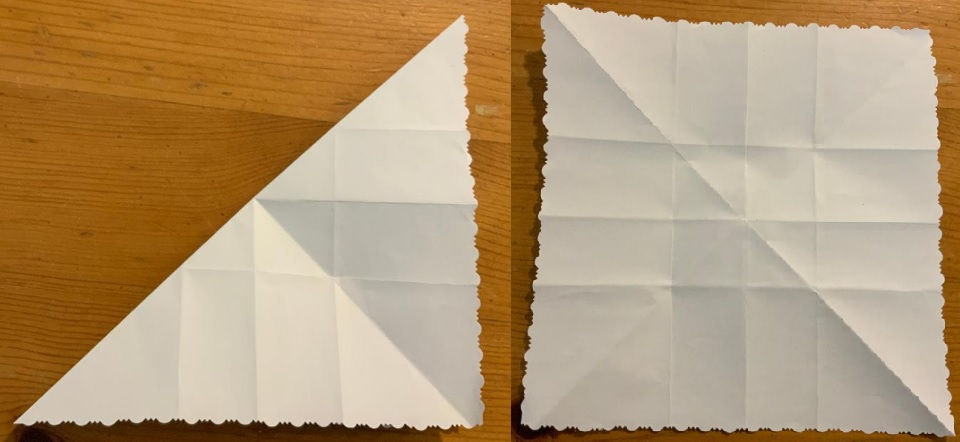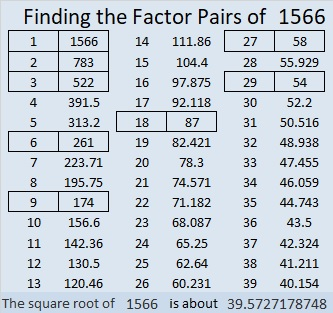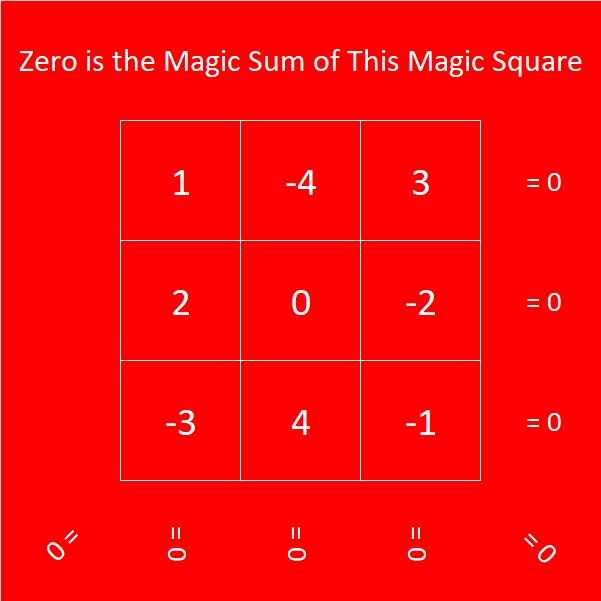# 1566 How to Make a Magic Square Snowflake

### Today’s Puzzle:

Can you place all the numbers from one to nine in a 3 × 3 grid so that the numbers on each of the four edges and the numbers on each of the four lines of symmetry (where the fold lines are) have the same sum?Here are some hints to get you started:

Begin by grouping and adding the numbers in this fashion:
Odd numbers 1 + 9 = 10,
Even numbers 2 + 8 = 10.
Odd numbers 3 + 7 = 10,
Even numbers 4 + 6 = 10, leaving you with just
5, which has no partner so it must go in the center of the magic square.

Those four sums plus 5 will form the four lines of symmetry, each with a magic sum of 10 + 5 = 15. Where do you put the sums in the magic square? Notice that the only way the edges can equal an odd number like 15 is to add two even numbers and one odd. (Adding two odd numbers and an even will never give you an odd number.) That means even numbers must go in the corners and an odd number will go between them.

Place the 1 in the middle of any edge. Place the nine in the middle on the opposite edge. What numbers will go on the edge with the 1? They must be two numbers that add up to 15 – 1 = 14. Only two of the even numbers add up to 14. Place them on the square on either side of the 1. Place their partners from the sums to 10 that are written above on their same lines of symmetry. Now you only have two odd numbers left to place. Place them so that those edges add up to 15.

Using a hole punch to make the numbers instead of writing numerals makes the snowflake even better: There are eight different orientations for the numbers to be on the snowflake, but all eight ways really only form one possible snowflake.### How to Make Your Own Magic Square Snowflake:

You will need an 8 1/2 by 11 sheet of white printer paper, scissors, and a hole punch. Decorative scissors are optional.

1. Begin with an 8 1/2 by 11 sheet of white printer paper. Follow  Paula Krieg’s instructions on how to fold and cut it into an 8 1/2 × 8 1/2 perfect square.
2. Make a crease along both diagonals of your square. Unfold.3. Follow EZ Origami’s instructions on how to fold your square into thirds. Unfold.
4. Turn your square 90° and follow EZ Origami’s instructions again to divide your square into thirds in the other direction.5. Fold snowflake in half vertically and then horizontally. Unfold.6. If desired, use decorative scissors to give your snowflake a pretty edge. You can cut two edges at the same time. Unfold.7. Make a narrow cut along the folds of the smaller square edges, taking care not to cut where the folds intersect. Unfold. (Do not use decorative scissors for this step.)8. Use the grid with numerals that you made earlier and a hole punch to place the right number of holes in each of the nine squares. An ordinary hole punch with handles will work, but I used the decorative one pictured below.I used the following facts to make my holes with my hole punch in the appropriate squares. Look at the snowflake picture above if clarification is needed:
1. Make a single punch in the center of its square.
2(1) = 2. Fold paper on diagonal, make a single punch above the diagonal.
2(3(1/2)) = 3. Fold paper on diagonal, make three half punches on the diagonal. Unfold.
2(2) = 4. Fold paper on diagonal, make two punches above the diagonal. Unfold.
4(1 + 1/4) = 5. The center square has four smaller squares. Fold center square to make one small square with four thicknesses, make 1/4 punch at corner of folds in center of snowflake. Make a single punch in center of small, thick square. Unfold.
2(3) = 6. Fold paper on diagonal, make three punches above the diagonal. Unfold.
2(3 + 1/2) = 7. Fold paper on diagonal, make half punch on center of diagonal and three punches above the diagonal. Unfold.
2(4) = 8. Fold paper on diagonal, make four punches above the diagonal. Unfold.
2((3 + 3(1/2)) = 9. Fold paper on diagonal, make three half punches on diagonal and three punches above the diagonal. Unfold.### Finding the Prime Factorization of 1566:

Since this is my 1566th post, I’ll explain how I find its prime factorization using as few divisions as I possibly can.

I know that 1566 can be divided by 9 because 1 + 5 + 6 + 6 = 18, a multiple of 9.
1566 ÷ 9 = 174.

I know that 174 can be divided by 6 because it is even and 1 + 7 + 4 = 12, a multiple of 3.
174 ÷ 6 = 29.

I show my work here:Now all that’s left to do is put the prime factors in numerical order with exponents.

### Factors of 1566:

• 1566 is a composite number.
• Prime factorization: 1566 = 2 × 3 × 3 × 3 × 29, which can be written 1566 = 2 × 3³ × 29.
• 1566 has at least one exponent greater than 1 in its prime factorization so √1566 can be simplified. Taking the factor pair from the factor pair table below with the largest square number factor, we get √1566 = (√9)(√174) = 3√174.
• The exponents in the prime factorization are 1,3 and 1. Adding one to each exponent and multiplying we get (1 + 1)(3 + 1)(1 + 1) = 2 × 4 × 2 = 16. Therefore 1566 has exactly 16 factors.
• The factors of 1566 are outlined with their factor pair partners in the graphic below.### More about the Number 1566:

1566 is the hypotenuse of a Pythagorean triple:
1080-1134-1566 which is (20-21-29) times 54.

Since 1566 is a multiple of 3, it is the magic sum of this 3 × 3 magic square:That can happen for ANY multiple of 3. Multiples of 3 that are less than 15 must have negative numbers in their magic squares in order to be the magic sum. For example, zero is a multiple of 3 because 3 × 0 = 0. Here’s how zero is the magic sum of a magic square: